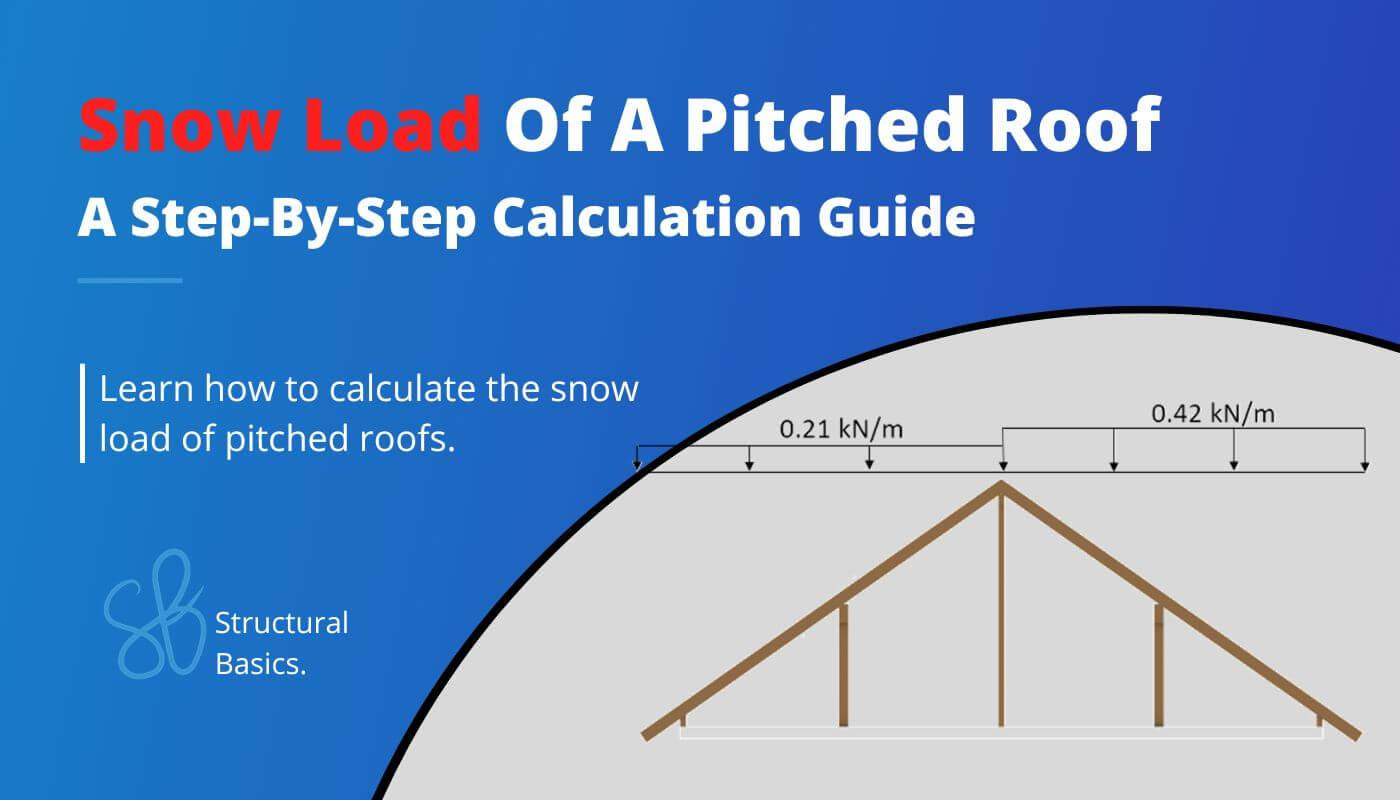# Snow Load Calculation Of Pitched Roofs {Step-By-Step Guide}Last updated: April 30th, 2023

In this blog post we will show how the characteristic snow load of a pitched roof is calculated according to Eurocode. Pitched roofs are for example purlin, rafter or collar beam roofs.

The calculation of the snow load for a pitched roof is a bit more complex then for a flat roof but we only need to follow 6 steps.

1. We first have to find the snow load shape coefficient $\mu_{1}$
2. Then the Exposure coefficient $C_{e}$
3. Thermal coefficient $C_{t}$
4. The characteristic snow load value on the ground $s_{k}$
5. Snow load value on the roof
6. In the last step, we have to create 3 cases with different load considerations on the left and right pitch.

A persistent / tansient design situation EN 1991-1-3 (5.1) is used to calculate the characteristic snow load:

$s = \mu_{i} * C_{e} * C_{t} * s_{k}$

Where

$\mu_{i}$ represents the snow load shape coefficient
$C_{e}$ represents the exposure coefficient
$C_{t}$ represents the thermal coefficient and
$s_{k}$ represents the characteristic snow load value on the ground

Let’s have a closer look how we get those parameters.

## Snow load shape coefficient $\mu_{1}$

The purlin roof we are looking at in this example counts as a pitched roof.

When increasing the angle of the roof slope over 30°, the snow load shape coefficient $\mu_{1}$ can be decreased below 0.8 due to sliding of the snow off the roof.

The value for $\mu_{1}$ is given in EN 1991-1-3 Table 5.2. For $\alpha$ = 40 which means that the slope of the roof is 40°, we get:

$\mu_{1} = 0.8 * (60 – \alpha)/30$
$\mu_{1} = 0.8 * (60 – 40)/30 = 0.53$

A slope of 40° reduces $\mu_{1}$ therefore by $\frac{0.533-0.8}{0.8}*100 = -33.4%$

## Exposure coefficient $C_{e}$

EN 1991-1-3 5.2 (7) recommends $C_{e}$ to be taken as 1.0. However this value depends on the topography of the location. EN 1991-1-3 Table 5.1 categorizes the topography in windswept, normal and sheltered with different values for $C_{e}$. In this blog post we assume a normal topography for our design. Therefore

$C_{e} = 1.0$

## Thermal coefficient $C_{t}$

EN 1991-1-3 5.2 (8) defines $C_{t}$ as 1.0.

However, this value can be reduced if the roof is covered by glass, which would lead to melting of the snow. In our case, we are not using any glass.

Therefore

$C_{t} = 1.0$

## Characteristic snow load value on the ground $s_{k}$

The characteristic snow load on the ground is found in the national annex of the country your roof is located in.

However, there is a great online tool which calculates the snow load according to location and national annex.

Click on the link, enter the location and the national annex.

If we do that for Copenhagen, Denmark, we get a value of

$s_{k} = 1.0 \frac{kN}{m^2}$

So now we finally have all 4 values required to calculate the characteristic snow load of a pitched roof (40° slope).

The snow load for this pitched roof located in Copenhagen, Denmark is calculated as

$s = \mu_{i} * C_{e} * C_{t} * s_{k}$
$s = 0.53 * 1.0 * 1.0 * 1.0 \frac{kN}{m^2} = 0.53 \frac{kN}{m^2}$

This load is now applied vertically to the pitched roof.

But because the roof is pitched, there are 3 different cases that we need to consider due to drifted load arrangements (EN 1991-1-3 5.3.3 (3) + (4)).

Case 1: s = 0.53 kN/m2 is applied on the whole area

Transformed in a 2d statical system we calculate the line load (kN/m) from the area load (kN/m2) by multiplying the area load with the spacing of the rafters = 0.8m.

$s = 0.53 \frac{kN}{m^2} * 0.8m = 0.42 \frac{kN}{m}$

Case 2: s = 0.53 kN/m2 is applied on the left slope and s/2 = 0.27 kN/m2 on the right slope

Transformed in a 2d statical system we calculate s/2 as

$s = \frac{0.42}{2} \frac{kN}{m} = 0.21 \frac{kN}{m}$

Case 3: s/2 = 0.27 kN/m2 is applied on the left slope and s = 0.53 kN/m2 on the right slope

Those 3 load cases need to be accounted for separately when doing the load combinations.

This means that every load combination which includes the snow load needs to be executed 3 times for the 3 different cases.

I know this is hard to understand in words, let’s look at an example.

We are picking out LC8 from our Load combination article from the ULS combinations.

LC8 needs to be executed 3 times now leading to

We are assuming the following values for the parameters. Check out the article if you want to know why.

which leads us to the following values of the 3 cases of LC8.

Now let’s apply the line loads to our statical system.